# NCERT Solutions for Class 12 Chemistry Chapter 8 The d and f Block Elements PDF - eSaral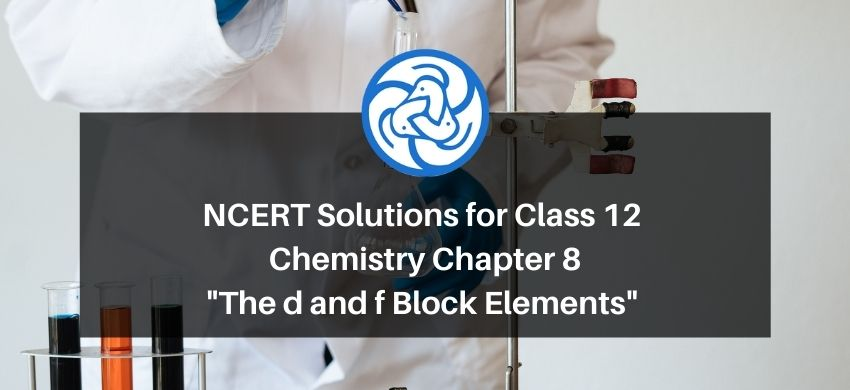# NCERT Solutions for Class 12 Chemistry Chapter 8 The d and f Block Elements PDF

Hey, are you a class 12 student and looking for ways to download NCERT Solutions for Class 12 Chemistry Chapter 8 The d and f Block Elements PDF? If yes. Then read this post till the end.

In this article, we have listed NCERT Solutions for Class 12 Chemistry Chapter 8 The d and f Block Elements in PDF that are prepared by Kota’s top IITian Faculties by keeping Simplicity in mind.

If you want to learn and understand class 12 Chemistry Chapter 8 "The d and f Block Elements" in an easy way then you can use these solutions PDF.

NCERT Solutions helps students to Practice important concepts of subjects easily. Class 12 Chemistry solutions provide detailed explanations of all the NCERT questions that students can use to clear their doubts instantly.

If you want to score high in your class 12 Chemistry Exam then it is very important for you to have a good knowledge of all the important topics, so to learn and practice those topics you can use eSaral NCERT Solutions.

In this article, we have listed NCERT Solutions for Class 12 Chemistry Chapter 8 The d and f Block Elements PDF that you can download to start your preparations anytime.

So, without wasting more time Let’s start.

### Download NCERT Solutions for Class 12 Chemistry Chapter 8 The d and f Block Elements PDF

Question 1: Silver atom has completely filled $d$ orbitals $\left(4 d^{10}\right)$ in its ground state. How can you say that it is a transition element?

Solution. Ag has a completely filled $4 d$ orbital $\left(4 d^{10} 5 s^{1}\right)$ in its ground state. Now, silver displays two oxidation states $(+1$ and +2). In the $+1$ oxidation state, an electron is removed from the s-orboital. However, in the $+2$ oxidation state, an electron is removed from the $d$-orbital. Thus, the $d$-orbital now becomes incomplete $\left(4 d^{9}\right)$. Hence, it is a transition element.

Question 2: In the series $\operatorname{Sc}(Z=21)$ to $\operatorname{Zn}(Z=30)$, the enthalpy of atomization of zinc is the lowest, i.e., $126 \mathrm{~kJ} \mathrm{~mol}^{-1}$. Why?

Solution. The extent of metallic bonding an element undergoes decides the enthalpy of atomization. The more extensive the metallic bonding of an element, the more will be its enthalpy of atomization. In all transition metals (except $\mathrm{Zn}$, electronic configuration: $\left.3 d^{10} 4 s^{2}\right)$, there are some unpaired electrons that account for their stronger metallic bonding Due to the absence of these unpaired electrons, the inter-atomic electronic bonding is the weakest in $\mathrm{Zn}$ and as a result, it has the least enthalpy of atomization.

Question 3: Which of the 3d series of the transition metals exhibits the largest number of oxidation states and why?

Solution. $\mathrm{Mn}(\mathrm{Z}=25)=3 d^{\circ} 4 s^{2}$

Mn has the maximum number of unpaired electrons present in the d-subshell (5 electrons). Hence, Mn exhibits the largest number of oxidation states, ranging from $+2$ to $+7$.

Question 4: The $E^{\theta}\left(\mathrm{M}^{2+} / \mathrm{M}\right)$ value for copper is positive $(+0.34 \mathrm{~V}) .$ What is possibly the reason for this? (Hint: consider its high $\Delta_{\mathrm{a}} H^{\theta}$ and low $\left.\Delta_{\mathrm{hyd}} H^{\theta}\right)$

Solution. The $E^{\theta}\left(\mathrm{M}^{2+} / \mathrm{M}\right)$ value of a metal depends on the energy changes involved in the following:

1. Sublimation: The energy required for converting one mole of an atom from the solid state to the gaseous state.

$\mathrm{M}_{(s)} \longrightarrow \mathrm{M}_{(g)} \quad \Delta_{\mathrm{s}} H($ Sublimation energy $)$

2. Ionization: The energy required to take out electrons from one mole of atoms in the gaseous state.

$\mathrm{M}_{(g)} \longrightarrow \mathrm{M}_{(g)}^{2+} \quad \Delta_{i} H$ (Ionization energy)

3. Hydration: The energy released when one mole of ions are hydrated.

$\mathrm{M}_{(g)}^{2+} \longrightarrow \mathrm{M}^{2+}{ }_{(a q)} \quad \Delta_{\mathrm{hyd}} H($ Hydration energy $)$

Now, copper has a high energy of atomization and low hydration energy. Hence, the $E^{\theta}\left(\mathrm{M}^{2+} / \mathrm{M}\right)$ value for copper is positive.

Question 5: How would you account for the irregular variation of ionization enthalpies (first and second) in the first series of the transition elements?

Solution. Ionization enthalpies are found to increase in the given series due to a continuous filling of the inner d-orbitals. The irregular variations of ionization enthalpies can be attributed to the extra stability of configurations such as $d^{0}, d^{5}, d^{10} .$ Since these states are exceptionally stable, their ionization enthalpies are very high.

In case of first ionization energy, $\mathrm{Cr}$ has low ionization energy. This is because after losing one electron, it attains the stable configuration $\left(3 d^{5}\right)$. On the other hand, $\mathrm{Zn}$ has exceptionally high first ionization energy as an electron has to be removed from stable and fully-filled orbitals $\left(3 d^{10} 4 s^{2}\right)$.

Second ionization energies are higher than the first since it becomes difficult to remove an electron when an electron has already been taken out. Also, elements like Cr and Cu have exceptionally high second ionization energies as after losing the first electron, they have attained the stable configuration $\left(\mathrm{Cr}^{+}: 3 d^{5}\right.$ and $\left.\mathrm{Cu}^{+}: 3 d^{10}\right)$. Hence, taking out one electron more from this stable configuration will require a lot of energy.

Question 6: Why is the highest oxidation state of a metal exhibited in its oxide or fluoride only?

Solution. Both oxide and fluoride ions are highly electronegative and have a very small size. Due to these properties, they are able to oxidize the metal to its highest oxidation state.

Question 7: Which is a stronger reducing agent $\mathrm{Cr}^{2+}$ or $\mathrm{Fe}^{2+}$ and why?

Solution. The following reactions are involved when $\mathrm{Cr}^{2+}$ and $\mathrm{Fe}^{2+}$ act as reducing agents.

$\mathrm{Cr}^{2+} \longrightarrow \mathrm{Cr}^{3+} \mathrm{Fe}^{2+} \longrightarrow \mathrm{Fe}^{3+}$

The $E_{\mathrm{Cr}^{4} / \mathrm{Cr}^{2}}^{\mathrm{2}}$ value is $-0.41 \mathrm{~V}$ and $E_{\mathrm{fe}^{4} / \mathrm{Fe}^{2}}^{\circ}$ is $+0.77 \mathrm{~V}$. This means that $\mathrm{Cr}^{2+}$ can be easily oxidized

to $\mathrm{Cr}^{3+}$, but $\mathrm{Fe}^{2+}$ does not get oxidized to $\mathrm{Fe}^{3+}$ easily. Therefore, $\mathrm{Cr}^{2+}$ is a better reducing agent that $\mathrm{Fe}^{3+}$

Question 8: Calculate the 'spin only' magnetic moment of $\mathrm{M}^{2+}{ }_{(a q)}$ ion $(Z=27)$.

Solution. $Z=27$

$\Rightarrow[\mathrm{Ar}] 3 d^{7} 4 s^{2}$

$\therefore \mathrm{M}^{2+}=[\mathrm{Ar}] 3 d^{7}$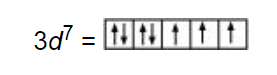i.e., 3 unpaired electrons

$\therefore n=3$

$\Rightarrow \sqrt{n(n+2)}=\mu$

$\Rightarrow \sqrt{3(3+2)}=\mu$

$\Rightarrow \sqrt{15}=\mu$

$\mu \approx 4 \mathrm{BM}$

Question 9: Explain why $\mathrm{Cu}^{+}$ion is not stable in aqueous solutions?

Solution. In an aqueous medium, $\mathrm{Cu}^{2+}$ is more stable than $\mathrm{Cu}^{+}$. This is because although energy is required to remove one electron from $\mathrm{Cu}^{+}$to $\mathrm{Cu}^{2+}$, high hydration energy of Cu2+ compensates for it. Therefore, $\mathrm{Cu}^{+}$ion in an aqueous solution is unstable. It disproportionates to give $\mathrm{Cu}^{2+}$ and $\mathrm{Cu}$.

$2 \mathrm{Cu}_{(a q)}+\mathrm{Cu}^{2+}{ }_{(a q)}+\mathrm{Cu}_{(s)}$

Question 10: Actinoid contraction is greater from element to element than lanthanoid contraction. Why?

Solution. In actinoids, $5 f$ orbitals are filled. These $5 f$ orbitals have a poorer shielding effect than $4 f$ orbitals (in lanthanoids). Thus, the effective nuclear charge experienced by electrons in valence shells in case of actinoids is much more that that experienced by lanthanoids. Hence, the size contraction in actinoids is greater as compared to that in lanthanoids.

Question 1: Write down the electronic configuration of:

(i) $\mathrm{Cr}^{3+}+\left(\right.$ iii) $\mathrm{Cu}^{+}(\mathrm{v}) \mathrm{Co}^{2}+(\mathrm{vii}) \mathrm{Mn}^{2+}$ (ii) $\mathrm{Pm}^{3+}(\mathrm{iv}) \mathrm{Ce}^{4+}(\mathrm{vi}) \mathrm{Lu}^{2+}(\mathrm{viii}) \mathrm{Th}^{4+}$

Solution. (i) $\mathrm{Cr}^{3+}: 1 s^{2} 2 s^{2} 2 p^{6} 3 s^{2} 3 p^{6} 3 d^{3}$

Or, $[\mathrm{Ar}]^{18} 3 d^{3}$

(ii) $\mathrm{Pm}^{3+}: 1 s^{2} 2 s^{2} 2 p^{6} 3 s^{2} 3 p^{6} 3 d^{10} 4 s^{2} 4 p^{6} 4 d^{10} 5 s^{2} 5 p^{6} 4 f^{4}$

Or, $[\mathrm{Xe}]^{54} 3 d^{3}$

(iii) $\mathrm{Cu}^{+}: 1 s^{2} 2 s^{2} 2 p^{6} 3 s^{2} 3 p^{6} 3 d^{10}$

Or, $[\mathrm{Ar}]^{18} 3 d^{10}$

(iv) $\mathrm{Ce}^{4+}: 1 s^{2} 2 s^{2} 2 p^{6} 3 s^{2} 3 p^{6} 3 d^{10} 4 s^{2} 4 p^{6} 4 d^{10} 5 s^{2} 5 p^{6}$

Or, $[\mathrm{Xe}]^{54}$

(v) $\mathrm{Co}^{2+}: 1 s^{2} 2 s^{2} 2 p^{6} 3 s^{2} 3 p^{6} 3 d^{7}$

Or, $[\mathrm{Ar}]^{18} 3 d^{7}$

(vi) $L u^{2+}: 1 s^{2} 2 s^{2} 2 p^{6} 3 s^{2} 3 p^{6} 3 d^{10} 4 s^{2} 4 p^{6} 4 d^{10} 5 s^{2} 5 p^{6} 4 f^{14} 5 d^{1}$

Or, $[\mathrm{Xe}]^{54} 2 f^{14} 3 d^{3}$

(vii) $\mathrm{Mn}^{2+}: 1 s^{2} 2 s^{2} 2 p^{6} 3 s^{2} 3 p^{6} 3 d^{5}$

Or, $[A r]^{18} 3 d^{5}$

(viii) $\mathrm{Th}^{4+}: 1 s^{2} 2 s^{2} 2 p^{6} 3 s^{2} 3 p^{6} 3 d^{10} 4 s^{2} 4 p^{6} 4 d^{10} 4 f^{14} 5 s^{2} 5 p^{6} 5 d^{10} 6 s^{2} 6 s^{6}$

Or, $[\mathrm{Rn}]^{86}$

Question 2: Why are $\mathrm{Mn}^{2+}$ compounds more stable than $\mathrm{Fe}^{2+}$ towards oxidation to their $+3$ state?

Solution. Electronic configuration of $\mathrm{Mn}^{2+}$ is $[\mathrm{Ar}]^{18} 3 d^{5}$.

Electronic configuration of $\mathrm{Fe}^{2+}$ is $[\mathrm{Ar}]^{18} 3 d^{6}$.

It is known that half-filled and fully-filled orbitals are more stable. Therefore, Mn in $(+2)$ state has a stable $d^{5}$ configuration. This is the reason $\mathrm{Mn}^{2+}$ shows resistance to oxidation to $\mathrm{Mn}^{3+}$. Also, $\mathrm{Fe}^{2+}$ has $3 d^{6}$ configuration and by losing one electron, its configuration changes to a more stable $3 d^{5}$ configuration. Therefore, $\mathrm{Fe}^{2+}$ easily gets oxidized to $\mathrm{Fe}^{+3}$ oxidation state

Question 3: Explain briefly how $+2$ state becomes more and more stable in the first half

of the first row transition elements with increasing atomic number?

Solution. The oxidation states displayed by the first half of the first row of transition metals are given in the table below.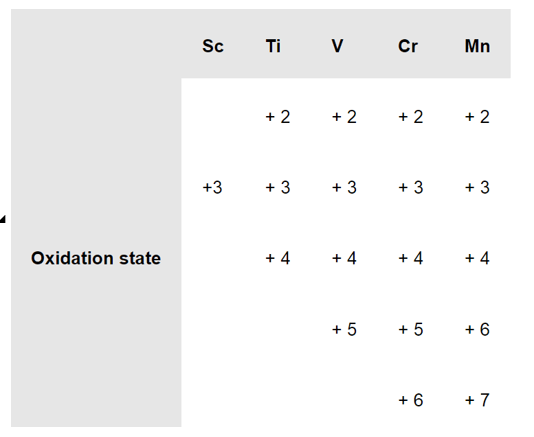It can be easily observed that except $\mathrm{Sc}$, all others metals display $+2$ oxidation state. Also, on moving from $\mathrm{Sc}$ to $\mathrm{Mn}$, the atomic number increases from 21 to 25 . This means the number of electrons in the $3 d$-orbital also increases from 1 to 5 .

$\operatorname{Sc}(+2)=d^{1}$

$\mathrm{Ti}(+2)=d^{2}$

$V(+2)=d^{3}$

$\operatorname{cr}(+2)=d^{4}$

$\operatorname{Mn}(+2)=d^{5}$

+2 oxidation state is attained by the loss of the two $4 s$ electrons by these metals. Since the number of $d$ electrons in $(+2)$ state also increases from $\mathrm{Ti}(+2)$ to $\mathrm{Mn}(+2)$, the stability of $+2$ state increases (as d-orbital is becoming more and more half-filled). Mn (+2) has $d^{5}$ electrons (that is half-filled $d$ shell, which is highly stable).

Question 4: To what extent do the electronic configurations decide the stability of oxidation states in the first series of the transition elements? Illustrate

Solution. The elements in the first-half of the transition series exhibit many oxidation states with Mn exhibiting maximum number of oxidation states $(+2$ to $+7)$. The stability of $+2$ oxidation state increases with the increase in atomic number. This happens as more electrons are getting filled in the $d$-orbital. However, Sc does not show +2 oxidation state. Its electronic configuration is $4 s^{2} 3 d^{1}$. It loses all the three electrons to form $\mathrm{Sc}^{3+}$. $+3$ oxidation state of $\mathrm{Sc}$ is very stable as by losing all three electrons, it attains stable noble gas configuration, [Ar]. Ti $(+4)$ and $V(+5)$ are very stable for the same reason. For Mn, $+2$ oxidation state is very stable as after losing two electrons, its $d$-orbital is exactly halffilled, $[\mathrm{Ar}] 3 d^{5}$

Question 5: What may be the stable oxidation state of the transition element with the

following $d$ electron configurations in the ground state of their atoms : $3 d^{3}$,

$3 d^{5}, 3 d^{8}$ and $3 d^{4} ?$

Solution.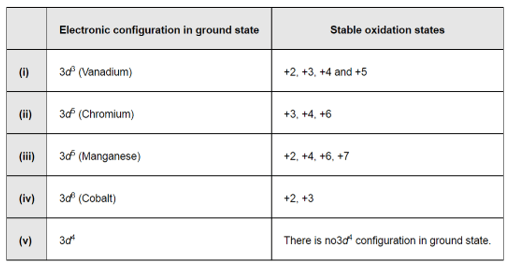Question 6: Name the oxometal anions of the first series of the transition metals in

which the metal exhibits the oxidation state equal to its group number.

Solution. (i) Vanadate, $\mathrm{VO}_{3}^{-}$

Oxidation state of $V$ is $+5$

(ii) Chromate, $\mathrm{CrO}_{4}^{2-}$

Oxidation state of $\mathrm{Cr}$ is $+6$.

(iii) Permanganate, $\mathrm{MnO}_{4}^{-}$

Oxidation state of $\mathrm{Mn}$ is $+7$.

Question 7: What is lanthanoid contraction? What are the consequences of lanthanoid contraction?

Solution. As we move along the lanthanoid series, the atomic number increases gradually by one. This means that the number of electrons and protons present in an atom also increases by one. As electrons are being added to the same shell, the effective nuclear charge increases. This happens because the increase in nuclear attraction due to the addition of proton is more pronounced than the increase in the interelectronic repulsions due to the addition of electron. Also, with the increase in atomic number, the number of electrons in the $4 f$ orbital also increases. The $4 f$ electrons have poor shielding effect. Therefore, the effective nuclear charge experienced by the outer electrons increases. Consequently, the attraction of the nucleus for the outermost electrons increases. This results in a steady decrease in the size of lanthanoids with the increase in the atomic number. This is termed as lanthanoid contraction.

Consequences of lanthanoid contraction

(i) There is similarity in the properties of second and third transition series.

ii. Separation of lanthanoids is possible due to lanthanide contraction.

(iii) It is due to lanithanide contraction that there is variation in the basic strength of lanthanide hydroxides. (Basic strength decreases from $\mathrm{La}(\mathrm{OH})_{3}$ to $\left.\mathrm{Lu}(\mathrm{OH})_{3} .\right)$

Question 8: What are the characteristics of the transition elements and why are they called transition elements? Which of the d-block elements may not be regarded as the transition elements?

Solution. Transition elements are those elements in which the atoms or ions (in stable oxidation state) contain partially filled d-orbital. These elements lie in the d-block and show a transition of properties between s-block and p-block. Therefore, these are called transition elements.

Elements such as Zn, Cd, and Hg cannot be classified as transition elements because these have completely filled d-subshell.

Question 9: In what way is the electronic configuration of the transition elements different that of the non-transition elements?

Solution. Transition metals have a partially filled $d$-orbital. Therefore, the electronic configuration of transition elements is (n 1) $d^{1-10} \mathrm{~ns}^{0-2}$.

The non-transition elements either do not have a d-orbital or have a fully filled $d$-orbital. Therefore, the electronic configuration of non-transition elements is $n s^{1-2}$ or $n s^{2} n p^{1-6}$.

Question 10: What are the different oxidation states exhibited by the lanthanoids?

Solution. In the lanthanide series, $+3$ oxidation state is most common i.e., $\operatorname{Ln}(I I I)$ compounds are predominant. However, $+2$ and +4 oxidation states can also be found in the solution or in solid compounds.

Question 11: Explain giving reasons:

(i) Transition metals and many of their compounds show paramagnetic

behaviour.

(ii) The enthalpies of atomisation of the transition metals are high.

(iii) The transition metals generally form coloured compounds.

(iv) Transition metals and their many compounds act as good catalyst.

Solution. (i) Transition metals show paramagnetic behaviour. Paramagnetism arises due to the presence of unpaired electrons with each electron having a magnetic moment associated with its spin angular momentum and orbital angular momentum. However, in the first transition series, the orbital angular momentum is quenched. Therefore, the resulting paramagnetism is only because of the unpaired electron.

(ii) Transition elements have high effective nuclear charge and a large number of valence electrons. Therefore, they form very strong metallic bonds. As a result, the enthalpy of atomization of transition metals is high.

(iii) Most of the complexes of transition metals are coloured. This is because of the absorption of radiation from visible light region to promote an electron from one of the d−orbitals to another. In the presence of ligands, the d-orbitals split up into two sets of orbitals having different energies. Therefore, the transition of electrons can take place from one set toanother. The energy required for these transitions is quite small and falls in the visible region of radiation. The ions of transition metals absorb the radiation of a particular wavelength and the rest is reflected, imparting colour to the solution.

(iv) The catalytic activity of the transition elements can be explained by two basic facts.

(a) Owing to their ability to show variable oxidation states and form complexes, transition metals form unstable intermediate compounds. Thus, they provide a new path with lower activation energy, $E_{\mathrm{a}}$, for the reaction.

(b) Transition metals also provide a suitable surface for the reactions to occur.

Question 12: What are interstitial compounds? Why are such compounds well known for transition metals?

Solution. Transition metals are large in size and contain lots of interstitial sites. Transition elements can trap atoms of other elements (that have small atomic size), such as $\mathrm{H}, \mathrm{C}, \mathrm{N}$, in the interstitial sites of their crystal lattices. The resulting compounds are called interstitial compounds.

Question 13: How is the variability in oxidation states of transition metals different from that of the non-transition metals? Illustrate with examples.

Solution. In transition elements, the oxidation state can vary from $+1$ to the highest oxidation state by removing all its valence electrons. Also, in transition elements, the oxidation states differ by $1\left(\mathrm{Fe}^{2+}\right.$ and $\mathrm{Fe}^{3+} ; \mathrm{Cu}^{+}$and $\left.\mathrm{Cu}^{2+}\right) .$ In non-transition elements, the oxidation states differ by 2, for example, $+2$ and $+4$ or $+3$ and $+5$, etc.

Question 14: Describe the preparation of potassium dichromate from iron chromite ore.

What is the effect of increasing pH on a solution of potassium dichromate?

Solution. Potassium dichromate is prepared from chromite ore $\left(\mathrm{FeCr}_{2} \mathrm{O}_{4}\right)$ in the following steps.

Step (1): Preparation of sodium chromate

$4 \mathrm{FeCr}_{2} \mathrm{O}_{4}+16 \mathrm{NaOH}+7 \mathrm{O}_{2} \longrightarrow 8 \mathrm{Na}_{2} \mathrm{CrO}_{4}+2 \mathrm{Fe}_{2} \mathrm{O}_{3}+8 \mathrm{H}_{2} \mathrm{O}$

Step (2): Conversion of sodium chromate into sodium dichromate

$2 \mathrm{Na}_{2} \mathrm{CrO}_{4}+\mathrm{conc} \cdot \mathrm{H}_{2} \mathrm{SO}_{4} \longrightarrow \mathrm{Na}_{2} \mathrm{Cr}_{2} \mathrm{O}_{7}+\mathrm{Na}_{2} \mathrm{SO}_{4}+\mathrm{H}_{2} \mathrm{O}$

Step(3): Conversion of sodium dichromate to potassium dichromate

$\mathrm{Na}_{2} \mathrm{Cr}_{2} \mathrm{O}_{7}+2 \mathrm{KCl} \longrightarrow \mathrm{K}_{2} \mathrm{Cr}_{2} \mathrm{O}_{7}+2 \mathrm{NaCl}$ Potassium dichromate being less soluble than sodium chloride is obtained in the form of orange coloured crystals and can be removed by filtration.

The dichromate ion $\left(\mathrm{Cr}_{2} \mathrm{O}_{7}^{2-}\right)$ exists in equilibrium with chromate $\left(\mathrm{CrO}_{4}^{2-}\right)$ ion at $\mathrm{pH} 4$. However, by changing the $\mathrm{pH}$, they can be interconverted.

$2 \mathrm{CrO}_{4}^{2-} \stackrel{\text { Acid }}{\longrightarrow} 2 \mathrm{HCrO}_{4}^{-} \stackrel{\text { Acid }}{\longrightarrow} 2 \mathrm{Cr}_{2} \mathrm{O}_{7}^{2-}$

$\mathrm{Cr}_{2} \mathrm{O}_{7}^{2-} \stackrel{\text { Base }}{\rightarrow} 2 \mathrm{HCrO}_{4}^{2-} \stackrel{\text { Base }}{\rightarrow} 2 \mathrm{CrO}_{4}^{2-}$

Question 15: Describe the oxidising action of potassium dichromate and write the ionic equations for its reaction with:

(i) iodide (ii) iron(II) solution and (iii) $\mathrm{H}_{2} \mathrm{~S}$

Solution. $\mathrm{K}_{2} \mathrm{Cr}_{2} \mathrm{O}_{7}$ acts as a very strong oxidising agent in the acidic medium.

$\mathrm{K}_{2} \mathrm{Cr}_{2} \mathrm{O}_{7}+4 \mathrm{H}_{2} \mathrm{SO}_{4} \longrightarrow \mathrm{K}_{2} \mathrm{SO}_{4}$

$+\mathrm{Cr}_{2}\left(\mathrm{SO}_{4}\right)_{3}+4 \mathrm{H}_{2} \mathrm{O}+3[\mathrm{O}]$

$\mathrm{K}_{2} \mathrm{Cr}_{2} \mathrm{O}_{7}$ takes up electrons to get reduced and acts as an oxidising agent. The reaction of $\mathrm{K}_{2} \mathrm{Cr}_{2} \mathrm{O}_{7}$ with other iodide, iron (II) solution, and $\mathrm{H}_{2} \mathrm{~S}$ are given below.

(i) $\mathrm{K}_{2} \mathrm{Cr}_{2} \mathrm{O}_{7}$ oxidizes iodide to iodine.

$\mathrm{Cr}_{2} \mathrm{O}_{7}^{2-}+14 \mathrm{H}^{+}+6 \mathrm{e}^{-} \longrightarrow 2 \mathrm{Cr}^{3+}+7 \mathrm{H}_{2} \mathrm{O}$

$\mathrm{Cr}_{2} \mathrm{O}_{7}^{2-}+14 \mathrm{H}^{+}+6 \mathrm{e}^{-} \longrightarrow 2 \mathrm{Cr}^{3+}+7 \mathrm{H}_{2} \mathrm{O}$

$\frac{ \left.2 \mathrm{I}^{\mathrm{-}} \longrightarrow \mathrm{I}_{2}+2 \mathrm{e}^{-}\right] \times 3}{\mathrm{Cr}_{2} \mathrm{O}_{7}^{2-}+6 \mathrm{I}^{-}+14 \mathrm{H}^{+} \longrightarrow 2 \mathrm{Cr}^{3}+3 \mathrm{I}_{2}+7 \mathrm{H}_{2} \mathrm{O}}$

(ii) $\mathrm{K}_{2} \mathrm{Cr}_{2} \mathrm{O}_{7}$ oxidizes iron (II) solution to iron (III) solution i.e., ferrous ions to ferric ions.

$\mathrm{Cr}_{2} \mathrm{O}_{7}^{2-}+14 \mathrm{H}^{+}+6 \mathrm{e}^{-} \longrightarrow 2 \mathrm{Cr}^{3+}+7 \mathrm{H}_{2} \mathrm{O}$

$\frac{ \left.\mathrm{Fe}^{2+} \longrightarrow \mathrm{Fe}^{3+}+\mathrm{e}^{-}\right] \times 6}{\mathrm{Cr}_{2} \mathrm{O}_{7}^{2-}+14 \mathrm{H}^{+}+6 \mathrm{Fe}^{2+} \longrightarrow 2 \mathrm{Cr}^{3+}+6 \mathrm{Fe}^{3+}+7 \mathrm{H}_{2} \mathrm{O}}$

(iii) $\mathrm{K}_{2} \mathrm{Cr}_{2} \mathrm{O}_{7}$ oxidizes $\mathrm{H}_{2} \mathrm{~S}$ to sulphur.

$\mathrm{Cr}_{2} \mathrm{O}_{7}^{2-}+14 \mathrm{H}^{+}+6 \mathrm{e}^{-} \longrightarrow 2 \mathrm{Cr}^{3+}+7 \mathrm{H}_{2} \mathrm{O}$

$\frac{ \left.\mathrm{H}_{2} \mathrm{~S} \longrightarrow \mathrm{S}+2 \mathrm{H}^{+}+2 \mathrm{e}^{-}\right] \times 3}{\mathrm{Cr}_{2} \mathrm{O}_{7}^{2-}+3 \mathrm{H}_{2} \mathrm{~S}+8 \mathrm{H}^{+} \longrightarrow 2 \mathrm{Cr}^{3+}+3 \mathrm{~S}+7 \mathrm{H}_{2} \mathrm{O}}$

Question 16: Describe the preparation of potassium permanganate. How does the acidified permanganate solution react with (i) iron(II) ions (ii) $\mathrm{SO}_{2}$ and (iii) oxalic acid?

Write the ionic equations for the reactions.

Solution. Potassium permanganate can be prepared from pyrolusite $\left(\mathrm{MnO}_{2}\right)$. The ore is fused with $\mathrm{KOH}$ in the presence of either atmospheric oxygen or an oxidising agent, such as $\mathrm{KNO}_{3}$ or $\mathrm{KClO}_{4}$, to give $\mathrm{K}_{2} \mathrm{MnO}_{4}$.

\begin{aligned} 2 \mathrm{MnO}_{2}+4 \mathrm{KOH}+\mathrm{O}_{2} \stackrel{\text { heat }}{\longrightarrow} & 2 \mathrm{~K}_{2} \mathrm{MnO}_{4}+2 \mathrm{H}_{2} \mathrm{O} \\ &(\text { Green }) \end{aligned}

The green mass can be extracted with water and then oxidized either electrolytically or by passing chlorine/ozone into the solution.

Electrolytic oxidation

$\mathrm{K}_{2} \mathrm{MnO}_{4} \longleftrightarrow 2 \mathrm{~K}^{+}+\mathrm{MnO}_{4}^{2-}$

$\mathrm{H}_{2} \mathrm{O} \longleftrightarrow \mathrm{H}^{+}+\mathrm{OH}^{-}$

At anode, manganate ions are oxidized to permanganate ions.

$\mathrm{MnO}_{4}^{2-} \longleftrightarrow \mathrm{MnO}_{4}^{-}+\mathrm{e}^{-}$

Green $\quad$ Purple

Oxidation by chlorine

$2 \mathrm{~K}_{2} \mathrm{MnO}_{4}+\mathrm{Cl}_{2} \longrightarrow 2 \mathrm{KMnO}_{4}+2 \mathrm{KCl}$

$2 \mathrm{MnO}_{4}^{2-}+\mathrm{Cl}_{2} \longrightarrow 2 \mathrm{MnO}_{4}^{-}+2 \mathrm{Cl}^{-}$

Oxidation by ozone

$2 \mathrm{~K}_{2} \mathrm{MnO}_{4}+\mathrm{O}_{3}+\mathrm{H}_{2} \mathrm{O}$

$\rightarrow 2 \mathrm{KMnO}_{4}+2 \mathrm{KOH}+\mathrm{O}_{2}$

$2 \mathrm{MnO}_{4}^{2-}+\mathrm{O}_{3}+\mathrm{H}_{2} \mathrm{O}$

$\longrightarrow 2 \mathrm{MnO}_{4}^{2-}+2 \mathrm{OH}^{-}+\mathrm{O}_{2}$

(i) Acidified $\mathrm{KMnO}_{4}$ solution oxidizes Fe (II) ions to Fe (III) ions i.e., ferrous ions to ferric ions.

$\mathrm{MnO}_{4}^{-}+8 \mathrm{H}^{+}+5 \mathrm{e}^{-} \longrightarrow \mathrm{Mn}^{2+}+4 \mathrm{H}_{2} \mathrm{O}$

$\frac{ \left.\mathrm{Fe}^{2+} \longrightarrow \mathrm{Fe}^{3+}+\mathrm{e}^{-}\right] \times 5}{\mathrm{MnO}_{4}^{-}+5 \mathrm{Fe}^{2+}+8 \mathrm{H}^{+} \longrightarrow \mathrm{Mn}^{2+}+5 \mathrm{Fe}^{3+}+4 \mathrm{H}_{2} \mathrm{O}}$

(ii) Acidified potassium permanganate oxidizes $\mathrm{SO}_{2}$ to sulphuric acid.

$\left.\mathrm{MnO}_{4}^{-}+6 \mathrm{H}^{+}+5 \mathrm{e}^{-} \longrightarrow \mathrm{Mn}^{2+}+3 \mathrm{H}_{2} \mathrm{O}\right] \times 2$

$\frac{ \left.2 \mathrm{H}_{2} \mathrm{O}+2 \mathrm{SO}_{2}+\mathrm{O}_{2} \longrightarrow 4 \mathrm{H}^{+}+2 \mathrm{SO}_{4}^{2-}+2 \mathrm{e}^{-}\right] \times 5}{2 \mathrm{MnO}_{4}^{-}+10 \mathrm{SO}_{2}+5 \mathrm{O}_{2}+4 \mathrm{H}_{2} \mathrm{O} \longrightarrow 2 \mathrm{Mn}^{2+}+10 \mathrm{SO}_{4}^{2-}+8 \mathrm{H}^{+}}$

(iii) Acidified potassium permanganate oxidizes oxalic acid to carbon dioxide.

$\left.\mathrm{MnO}_{4}^{-}+8 \mathrm{H}^{+}+5 \mathrm{e}^{-} \longrightarrow \mathrm{Mn}^{2+}+4 \mathrm{H}_{2} \mathrm{O}\right] \times 2$

$\frac{ \left.\mathrm{C}_{2} \mathrm{O}_{4}^{2-} \longrightarrow 2 \mathrm{CO}_{2}+2 \mathrm{e}^{-}\right] \times 5}{2 \mathrm{MnO}_{4}^{-}+5 \mathrm{C}_{2} \mathrm{O}_{4}^{2-}+16 \mathrm{H}^{+} \longrightarrow 2 \mathrm{Mn}^{2+}+10 \mathrm{CO}_{2}+8 \mathrm{H}_{2} \mathrm{O}}$

Question 17: For $\mathrm{M}^{2+} / \mathrm{M}$ and $\mathrm{M}^{3+} / \mathrm{M}^{2+}$ systems, the $\mathrm{E}^{\ominus}$ values for some metals are as follows:

$\mathrm{Cr}^{2+} / \mathrm{Cr}-0.9 \mathrm{~V}$

$\mathrm{Cr}^{3} / \mathrm{Cr}^{2+}-0.4 \mathrm{~V}$

$\mathrm{Mn}^{2+} / \mathrm{Mn}-1.2 \mathrm{~V}$

$\mathrm{Mn}^{3+} / \mathrm{Mn}^{2+}+1.5 \mathrm{~V}$

$\mathrm{Fe}^{2+} / \mathrm{Fe}-0.4 \mathrm{~V}$

$\mathrm{Fe}^{3+} / \mathrm{Fe}^{2+}+0.8 \mathrm{~V}$

Use this data to comment upon:

(i) The stability of $\mathrm{Fe}^{3+}$ in acid solution as compared to that of $\mathrm{Cr}^{3+}$ or $\mathrm{Mn}^{3+}$ and

(ii) The ease with which iron can be oxidised as compared to a similar process for either chromium or manganese metal.

Solution. (i) The ${ }^{\Theta}{ }^{\ominus}$ value for $\mathrm{Fe}^{3+} / \mathrm{Fe}^{2+}$ is higher than that for $\mathrm{Cr}^{3+} / \mathrm{Cr}^{2+}$ and lower than that for $\mathrm{Mn}^{3+} / \mathrm{Mn}^{2+} .$ So, the reduction of $\mathrm{Fe}^{3+}$ to $\mathrm{Fe}^{2+}$ is easier than the reduction of $\mathrm{Mn}^{3+}$ to $\mathrm{Mn}^{2+}$, but not as easy as the reduction of $\mathrm{Cr}^{3+}$ to $\mathrm{Cr}^{2+} .$ Hence, $\mathrm{Fe}^{3+}$ is more stable than $\mathrm{Mn}^{3+}$, but less stable than $\mathrm{Cr}^{3+}$. These metal ions can be arranged in the increasing order of their stability as: $\mathrm{Mn}^{3+}<\mathrm{Fe}^{3+}<\mathrm{Cr}^{3+}$

(ii) The reduction potentials for the given pairs increase in the following order.

$\mathrm{Mn}^{2+} / \mathrm{Mn}<\mathrm{Cr}^{2+} / \mathrm{Cr}<\mathrm{Fe}^{2+} / \mathrm{Fe}$

So, the oxidation of $\mathrm{Fe}$ to $\mathrm{Fe}^{2+}$ is not as easy as the oxidation of $\mathrm{Cr}$ to $\mathrm{Cr}^{2+}$ and the oxidation of $\mathrm{Mn}$ to $\mathrm{Mn}^{2+}$. Thus, these metals can be arranged in the increasing order of their ability to get oxidised as: $\mathrm{Fe}<\mathrm{Cr}<\mathrm{Mn}$

Question 18: Predict which of the following will be coloured in aqueous solution? $\mathrm{Ti}^{3+}, \mathrm{V}^{3+}$,

$\mathrm{Cu}^{+}, \mathrm{Sc}^{3+}, \mathrm{Mn}^{2+}, \mathrm{Fe}^{3+}$ and $\mathrm{Co}^{2+} .$ Give reasons for each.

Solution. Only the ions that have electrons in d-orbital and in which d-d transition is possible will be coloured. The ions in which d-orbitals are empty or completely filled will be colourless as no d-d transition is possible in those configurations.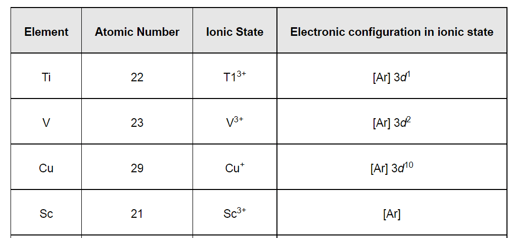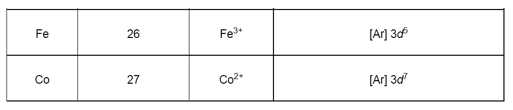From the above table, it can be easily observed that only $\mathrm{Sc}^{3+}$ has an empty $d$-orbital and $\mathrm{Cu}^{+}$has completely filled dorbitals. All other ions, except $\mathrm{Sc}^{3+}$ and $\mathrm{Cu}^{+}$, will be coloured in aqueous solution because of $d-d$ transitions.

Question 19: Compare the stability of $+2$ oxidation state for the elements of the first transition series.

Solution.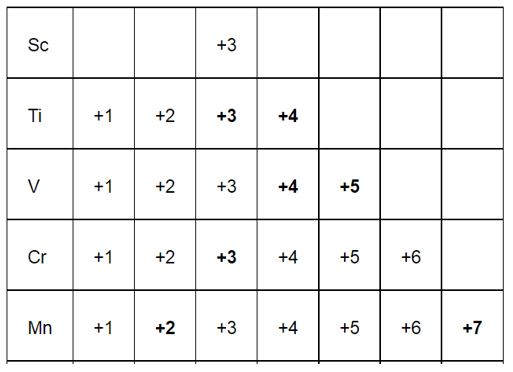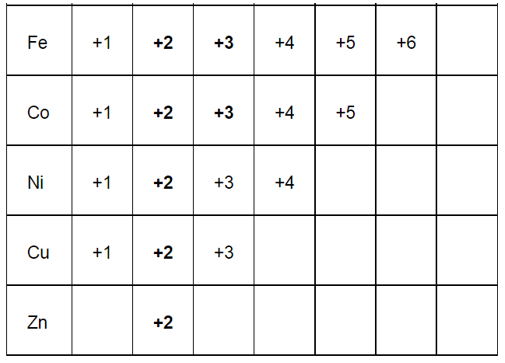From the above table, it is evident that the maximum number of oxidation states is shown by Mn, varying from $+2$ to +7. The number of oxidation states increases on moving from Sc to Mn. On moving from Mn to Zn, the number of oxidation states decreases due to a decrease in the number of available unpaired electrons. The relative stability of the $+2$ oxidation state increases on moving from top to bottom. This is because on moving from top to bottom, it becomes more and more difficult to remove the third electron from the $d$-orbital.

Question 20: Compare the chemistry of actinoids with that of the lanthanoids with special reference to: (i) electronic configuration

(ii) atomic and ionic sizes and

(iii) oxidation state

(iv) chemical reactivity.

Solution. (i) Electronic configuration

The general electronic configuration for lanthanoids is $[\mathrm{Xe}]^{54} 4 f^{0-14} 5 d^{0-1} 6 s^{2}$ and that for actinoids is $[\mathrm{Rn}]^{86} 5 f^{f-14} 6 d^{0-1}$ $7 s^{2}$. Unlike 4f orbitals, $5 f$ orbitals are not deeply buried and participate in bonding to a greater extent.

(ii) Oxidation states

The principal oxidation state of lanthanoids is (+3). However, sometimes we also encounter oxidation states of $+2$ and + 4 . This is because of extra stability of fully-filled and half-filled orbitals. Actinoids exhibit a greater range of oxidation states. This is because the $5 f, 6 d$, and $7 s$ levels are of comparable energies. Again, $(+3)$ is the principal oxidation state for actinoids. Actinoids such as lanthanoids have more compounds in $+3$ state than in $+4$ state.

(iii) Atomic and lonic sizes

Similar to lanthanoids, actinoids also exhibit actinoid contraction (overall decrease in atomic and ionic radii). The contraction is greater due to the poor shielding effect of $5 f$ orbitals.

iv. Chemical reactivity In the lanthanide series, the earlier members of the series are more reactive. They have reactivity that is comparable to Ca. With an increase in the atomic number, the lanthanides start behaving similar to Al. Actinoids, on the other hand, are highly reactive metals, especially when they are finely divided. When they are added to boiling water, they give a mixture of oxide and hydride. Actinoids combine with most of the non-metals at moderate temperatures. Alkalies have no action on these actinoids. In case of acids, they are slightly affected by nitric acid (because of the formation of a protective oxide layer).

Question 21: How would you account for the following: (i) Of the $d^{4}$ species, $\mathrm{Cr}^{2+}$ is strongly reducing while manganese(III) is strongly oxidising.

(ii) Cobalt(II) is stable in aqueous solution but in the presence of complexing reagents it is easily oxidised.

(iii) The $d^{1}$ configuration is very unstable in ions.

Solution. (i) $\mathrm{Cr}^{2+}$ is strongly reducing in nature. It has a $d^{4}$ configuration. While acting as a reducing agent, it gets oxidized to $\mathrm{Cr}^{3+}$ (electronic configuration, $\left.d^{3}\right)$. This $d^{\beta}$ configuration can be written as $t_{2 \mathrm{~g}}^{3}$ configuration, which is a more stable configuration. In the case of $\mathrm{Mn}^{3+}\left(d^{4}\right)$, it acts as an oxidizing agent and gets reduced to $\mathrm{Mn}^{2+}\left(d^{5}\right)$. This has an exactly half-filled $d$-orbital and is highly stable.

(ii) $\mathrm{Co}(\mathrm{II})$ is stable in aqueous solutions. However, in the presence of strong field complexing reagents, it is oxidized to Co (III). Although the $3^{\text {rd }}$ ionization energy for $\mathrm{Co}$ is high, but the higher amount of crystal field stabilization energy (CFSE) released in the presence of strong field ligands overcomes this ionization energy.

(iii) The ions in $d^{1}$ configuration tend to lose one more electron to get into stable $d^{0}$ configuration. Also, the hydration or lattice energy is more than sufficient to remove the only electron present in the d-orbital of these ions. Therefore, they act as reducing agents.

Question 22: What is meant by ‘disproportionation’? Give two examples of disproportionation reaction in aqueous solution.

Solution. It is found that sometimes a relatively less stable oxidation state undergoes an oxidation-reduction reaction in which it is simultaneously oxidised and reduced. This is called disproportionation.

For example,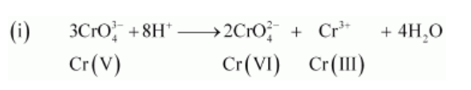$\operatorname{Cr}(\mathrm{V})$ is oxidized to $\operatorname{Cr}(\mathrm{VI})$ and reduced to $\mathrm{Cr}(\mathrm{III}) .$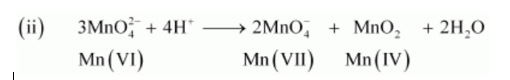Mn (VI) is oxidized to Mn (VII) and reduced to Mn (IV).

Question 23: Which metal in the first series of transition metals exhibits $+1$ oxidation state most frequently and why?

Solution. In the first transition series, Cu exhibits $+1$ oxidation state very frequently. It is because $\mathrm{Cu}(+1)$ has an electronic configuration of $[\mathrm{Ar}] 3 d^{10}$. The completely filled $d$-orbital makes it highly stable.

Question 24: Calculate the number of unpaired electrons in the following gaseous ions: $\mathrm{Mn}^{3+}, \mathrm{Cr}^{3+}, \mathrm{V}^{3+}$ and $\mathrm{Ti}^{3+}$. Which one of these is the most stable in aqueous solution?

Solution.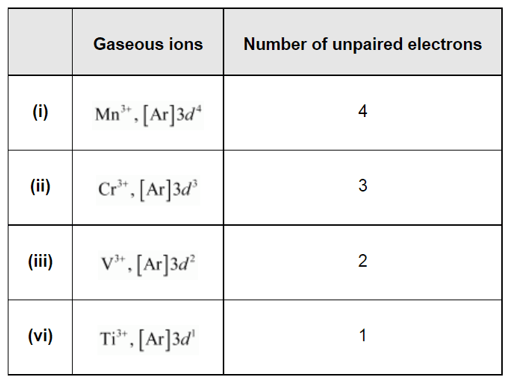$\mathrm{Cr}^{3+}$ is the most stable in aqueous solutions owing to a $t_{2 \mathrm{~g}}^{3}$ configuration.

Question 25: Give examples and suggest reasons for the following features of the transition metal chemistry:

(i)The lowest oxide of transition metal is basic, the highest is amphoteric/acidic.

(ii)A transition metal exhibits highest oxidation state in oxides and fluorides.

(iii) The highest oxidation state is exhibited in oxoanions of a metal.

Solution. (i) In the case of a lower oxide of a transition metal, the metal atom has a low oxidation state. This means that some of the valence electrons of the metal atom are not involved in bonding. As a result, it can donate electrons and behave as a base.

On the other hand, in the case of a higher oxide of a transition metal, the metal atom has a high oxidation state. This means that the valence electrons are involved in bonding and so, they are unavailable. There is also a high effective nuclear charge.

As a result, it can accept electrons and behave as an acid.

For example, $\mathrm{Mn}^{\mathrm{II}} \mathrm{O}$ is basic and $\mathrm{M} \mathrm{n}_{2} \mathrm{O}_{7}^{\mathrm{VII}}$ is acidic.

(ii) Oxygen and fluorine act as strong oxidising agents because of their high electronegativities and small sizes. Hence, they bring out the highest oxidation states from the transition metals. In other words, a transition metal exhibits higher oxidation states in oxides and fluorides. For example, in $\mathrm{OsF}_{6}$ and $\mathrm{V}_{2} \mathrm{O}_{5}$, the oxidation states of $\mathrm{Os}$ and $\mathrm{V}$ are $+6$ and $+5$ respectively.

(iii) Oxygen is a strong oxidising agent due to its high electronegativity and small size. So, oxo-anions of a metal have the highest oxidation state. For example, in $\mathrm{MnO}_{4}^{-}$, the oxidation state of Mn is $+7$.

Question 26: Indicate the steps in the preparation of:

(i) K2Cr2O7 from chromite ore.

(ii) KMnO4 from pyrolusite ore.

Solution. (i) Potassium dichromate $\left(\mathrm{K}_{2} \mathrm{Cr}_{2} \mathrm{O}_{7}\right)$ is prepared from chromite ore $\left(\mathrm{FeCr}_{2} \mathrm{O}_{4}\right)$ in the following steps.

Step (1): Preparation of sodium chromate

$4 \mathrm{FeCr}_{2} \mathrm{O}_{4}+16 \mathrm{NaOH}+7 \mathrm{O}_{2}$

$\longrightarrow 8 \mathrm{Na}_{2} \mathrm{CrO}_{4}+2 \mathrm{Fe}_{2} \mathrm{O}_{3}+8 \mathrm{H}_{2} \mathrm{O}$

Step (2): Conversion of sodium chromate into sodium dichromate

$2 \mathrm{Na}_{2} \mathrm{CrO}_{4}+\mathrm{conc} \cdot \mathrm{H}_{2} \mathrm{SO}_{4}$

$\longrightarrow \mathrm{Na}_{2} \mathrm{Cr}_{2} \mathrm{O}_{7}+\mathrm{Na}_{2} \mathrm{SO}_{4}+\mathrm{H}_{2} \mathrm{O}$

Step(3): Conversion of sodium dichromate to potassium dichromate

$\mathrm{Na}_{2} \mathrm{Cr}_{2} \mathrm{O}_{7}+2 \mathrm{KCl} \longrightarrow \mathrm{K}_{2} \mathrm{Cr}_{2} \mathrm{O}_{7}+2 \mathrm{NaCl}$

Potassium chloride being less soluble than sodium chloride is obtained in the form of orange coloured crystals and can be removed by filtration.

The dichromate ion $\left(\mathrm{Cr}_{2} \mathrm{O}_{7}^{2-}\right)$ exists in equilibrium with chromate $\left(\mathrm{CrO}_{4}^{2-}\right)$ ion at $\mathrm{pH} 4$. However, by changing the $\mathrm{pH}$, they can be interconverted.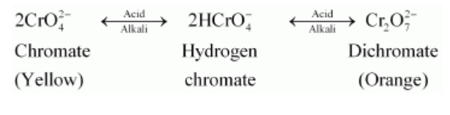(ii) Potassium permanganate $\left(\mathrm{KMnO}_{4}\right)$ can be prepared from pyrolusite $\left(\mathrm{MnO}_{2}\right)$. The ore is fused with $\mathrm{KOH}$ in the presence of either atmospheric oxygen or an oxidising agent, such as $\mathrm{KNO}_{3}$ or $\mathrm{KClO}_{4}$, to give $\mathrm{K}_{2} \mathrm{MnO}_{4}$.

\begin{aligned} 2 \mathrm{MnO}_{2}+4 \mathrm{KOH}+\mathrm{O}_{2} \stackrel{\text { heat }}{\longrightarrow} & 2 \mathrm{~K}_{2} \mathrm{MnO}_{4}+2 \mathrm{H}_{2} \mathrm{O} \\ &(\text { Green }) \end{aligned}

The green mass can be extracted with water and then oxidized either electrolytically or by passing chlorine/ozone into the solution.

Electrolytic oxidation

$\mathrm{K}_{2} \mathrm{MnO}_{4} \longleftrightarrow 2 \mathrm{~K}^{+}+\mathrm{MnO}_{4}^{2-}$

$\mathrm{H}_{2} \mathrm{O} \longleftrightarrow \mathrm{H}^{+}+\mathrm{OH}^{-}$

At anode, manganate ions are oxidized to permanganate ions.

$\mathrm{MnO}_{4}^{2-} \longleftrightarrow \mathrm{MnO}_{4}^{-}+\mathrm{e}^{-}$

Green $\quad$ Purple

Oxidation by chlorine

$2 \mathrm{~K}_{2} \mathrm{MnO}_{4}+\mathrm{Cl}_{2} \longrightarrow 2 \mathrm{KMnO}_{4}+2 \mathrm{KCl}$

$2 \mathrm{MnO}_{4}^{2-}+\mathrm{Cl}_{2} \longrightarrow 2 \mathrm{MnO}_{4}^{-}+2 \mathrm{Cl}^{-}$

Oxidation by ozone

$2 \mathrm{~K}_{2} \mathrm{MnO}_{4}+\mathrm{O}_{3}+\mathrm{H}_{2} \mathrm{O}$

$\longrightarrow 2 \mathrm{KMnO}_{4}+2 \mathrm{KOH}+\mathrm{O}_{2}$

$2 \mathrm{MnO}_{4}^{2-}+\mathrm{O}_{3}+\mathrm{H}_{2} \mathrm{O}$

$\longrightarrow 2 \mathrm{MnO}_{4}^{2-}+2 \mathrm{OH}^{-}+\mathrm{O}_{2}$

Question 27: What are alloys? Name an important alloy which contains some of the lanthanoid metals. Mention its uses.

Solution. An alloy is a solid solution of two or more elements in a metallic matrix. It can either be a partial solid solution or a complete solid solution. Alloys are usually found to possess different physical properties than those of the component elements.

An important alloy of lanthanoids is Mischmetal. It contains lanthanoids $(94-95 \%)$, iron $(5 \%)$, and traces of $S, C$, Si, $\mathrm{Ca}$, and $\mathrm{Al}$.

Uses

(1) Mischmetal is used in cigarettes and gas lighters.

(2) It is used in flame throwing tanks.

(3) It is used in tracer bullets and shells.

Question 28: What are inner transition elements? Decide which of the following atomic numbers are the atomic numbers of the inner transition elements: $29,59,74,95,102,104$.

Solution. Inner transition metals are those elements in which the last electron enters the $f$-orbital. The elements in which the $4 f$ and the 5 f orbitals are progressively filled are called $f$-block elements. Among the given atomic numbers, the atomic numbers of the inner transition elements are 59,95, and 102 .

Question 29: The chemistry of the actinoid elements is not so smooth as that of the Lanthanoids. Justify this statement by giving some examples from the oxidation state of these elements.

Solution. Lanthanoids primarily show three oxidation states $(+2,+3,+4)$. Among these oxidation states, $+3$ state is the most common. Lanthanoids display a limited number of oxidation states because the energy difference between $4 f, 5 d$, and 6s orbitals is quite large. On the other hand, the energy difference between $5 f, 6 d$, and $7 s$ orbitals is very less. Hence, actinoids display a large number of oxidation states. For example, uranium and plutonium display $+3,+4,+5$, and $+6$ oxidation states while neptunium displays $+3,+4,+5$, and $+7$. The most common oxidation state in case of actinoids is also $+3$.

Question 30: Which is the last element in the series of the actinoids? Write the electronic configuration of this element. Comment on the possible oxidation state of this element.

Solution. The last element in the actinoid series is lawrencium, Lr. Its atomic number is 103 and its electronic configuration is $[\mathrm{Rn}] 5 f^{14} 6 d^{1} 7 s^{2}$. The most common oxidation state displayed by it is $+3$; because after losing 3 electrons it attains stable $f^{14}$ configuration.

Question 31: Use Hund's rule to derive the electronic configuration of $\mathrm{Ce}^{3+}$ ion and calculate its magnetic moment on the basis of 'spin-only' formula.

Solution. Ce $: 1 s^{2} 2 s^{2} 2 p^{6} 3 s^{2} 3 p^{6} 3 d^{10} 4 s^{2} 4 p^{6} 4 d^{10} 5 s^{2} 5 p^{6} 4 f^{1} 5 d^{1} 6 s^{2}$

Magnetic moment can be calculated as:

$\mu=\sqrt{n(n+2)}$

Where,

n = number of unpaired electrons

The electronic configuration of $\mathrm{Ce}^{3+}$

$1 s^{2} 2 s^{2} 2 p^{6} 3 s^{2} 3 p^{6} 3 d^{10} 4 s^{2} 4 p^{6} 4 d^{10} 5 s^{2} 5 p^{6} 4 f^{1}$

$\ln \mathrm{Ce}^{3+}, n=1$

$\therefore \mu=\sqrt{1(1+2)}$

$=\sqrt{3}=1.732 \mathrm{~B} . \mathrm{M}$

Question 32: Name the members of the lanthanoid series which exhibit +4 oxidation state and those which exhibit +2 oxidation state. Try to correlate this type of behavior with the electronic configurations of these elements.

Solution. The lanthanides that exhibit $+2$ and $+4$ states are shown in the given table. The atomic numbers of the elements are given in the parenthesis.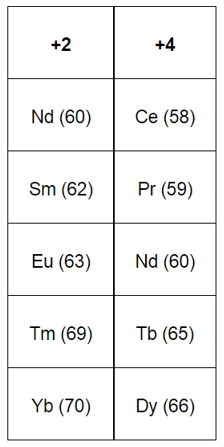Ce after forming $\mathrm{Ce}^{4+}$ attains a stable electronic configuration of [Xe].

Tb after forming $\mathrm{Tb}^{4+}$ attains a stable electronic configuration of [Xe] $4 f^{7}$.

Eu after forming $\mathrm{Eu}^{2+}$ attains a stable electronic configuration of $[\mathrm{Xe}] 4 f^{7}$.

Yb after forming $\mathrm{Yb}^{2+}$ attains a stable electronic configuration of $[\mathrm{Xe}] 4 f^{14} .$

Question 33: Compare the chemistry of the actinoids with that of lanthanoids with reference to:

(i) electronic configuration

(ii) oxidation states and

(iii) chemical reactivity.

Solution. Electronic configurationThe general electronic configuration for lanthanoids is $[\mathrm{Xe}]^{54} 4 f^{0-14} 5 d^{0-1} 6 s^{2}$ and that for actinoids is $[\mathrm{Rn}]^{86} 5 f^{1-14} 6 d^{0-1}$ $7 s^{2}$. Unlike $4 f$ orbitals, $5 f$ orbitals are not deeply buried and participate in bonding to a greater extent.

Oxidation states

The principal oxidation state of lanthanoids is (+3). However, sometimes we also encounter oxidation states of + 2 and + 4 . This is because of extra stability of fully-filled and half-filled orbitals. Actinoids exhibit a greater range of oxidation states. This is because the $5 f, 6 d$, and $7 s$ levels are of comparable energies. Again, $(+3)$ is the principal oxidation state for actinoids. Actinoids such as lanthanoids have more compounds in $+3$ state than in $+4$ state.

Chemical reactivity

In the lanthanide series, the earlier members of the series are more reactive. They have reactivity that is comparable to Ca. With an increase in the atomic number, the lanthanides start behaving similar to Al. Actinoids, on the other hand, are highly reactive metals, especially when they are finely divided. When they are added to boiling water, they give a mixture of oxide and hydride. Actinoids combine with most of the non-metals at moderate temperatures. Alkalies have no action on these actinoids. In case of acids, they are slightly affected by nitric acid (because of the formation of a protective oxide layer).

Question 34: Write the electronic configurations of the elements with the atomic numbers 61, 91, 101, and 109.

Solution.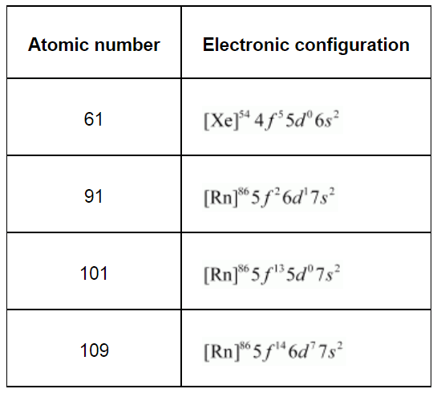Question 35: Compare the general characteristics of the first series of the transition metals with those of the second and third series metals in the respective vertical columns. Give special emphasis on the following points:

(i) electronic configurations,

(ii) oxidation states,

(iii) ionisation enthalpies, and

(iv) atomic sizes.

Solution. (i) In the $1^{\text {st }}, 2^{\text {nd }}$ and $3^{\text {rd }}$ transition series, the $3 d, 4 d$ and $5 d$ orbitals are respectively filled.

We know that elements in the same vertical column generally have similar electronic configurations.

In the first transition series, two elements show unusual electronic configurations:

$\operatorname{Cr}(24)=3 d^{5} 4 s^{1}$

$\mathrm{Cu}(29)=3 d^{10} 4 s^{\prime}$

Similarly, there are exceptions in the second transition series. These are:

Mo $(42)=4 d^{5} 5 s^{\prime}$

$\mathrm{Tc}(43)=4 d^{6} 5 s^{\prime}$

$\operatorname{Ru}(44)=4 d^{7} 5 s^{1}$

$\operatorname{Rh}(45)=4 d^{8} 5 s^{1}$

$\operatorname{Pd}(46)=4 d^{10} 5 s^{0}$

$\operatorname{Ag}(47)=4 d^{10} 5 s^{\prime}$

There are some exceptions in the third transition series as well. These are:

$\mathrm{W}(74)=5 d^{4} 6 s^{2}$

$\operatorname{Pt}(78)=5 d^{9} 6 s^{\prime}$

$\mathrm{Au}(79)=5 d^{10} 6 s^{\prime}$

As a result of these exceptions, it happens many times that the electronic configurations of the elements present in the same group are dissimilar.

(ii) In each of the three transition series the number of oxidation states shown by the elements is the maximum in the middle and the minimum at the extreme ends.

However, $+2$ and $+3$ oxidation states are quite stable for all elements present in the first transition series. All metals present in the first transition series form stable compounds in the $+2$ and $+3$ oxidation states. The stability of the $+2$ and $+3$ oxidation states decreases in the second and the third transition series, wherein higher oxidation states are more important.

For example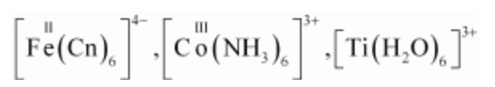are stable complexes, but no such complexes are known for the second and third transition series such as Mo, W, Rh, In. They form complexes in which their oxidation states are high. For example:

$\mathrm{WCl}_{6}, \mathrm{ReF}_{7}, \mathrm{RuO}_{4}$, etc.

(iii) In each of the three transition series, the first ionisation enthalpy increases from left to right. However, there are some exceptions. The first ionisation enthalpies of the third transition series are higher than those of the first and second transition series. This occurs due to the poor shielding effect of $4 f$ electrons in the third transition series.

Certain elements in the second transition series have higher first ionisation enthalpies than elements corresponding to the same vertical column in the first transition series. There are also elements in the $2^{\text {nd }}$ transition series whose first ionisation enthalpies are lower than those of the elements corresponding to the same vertical column in the $1^{\text {st }}$ transition series.

(iv) Atomic size generally decreases from left to right across a period. Now, among the three transition series, atomic sizes of the elements in the second transition series are greater than those of the elements corresponding to the same vertical column in the first transition series. However, the atomic sizes of the elements in the third transition series are virtually the same as those of the corresponding members in the second transition series. This is due to lanthanoid contraction.

Question 36: Write down the number of 3d electrons in each of the following ions:

$\mathrm{Ti}^{2+}, \mathrm{V}^{2+}, \mathrm{Cr}^{3+}, \mathrm{Mn}^{2+}, \mathrm{Fe}^{2+}, \mathrm{Fe}^{3+}, \mathrm{CO}^{2+}, \mathrm{Ni}^{2+}$ and $\mathrm{Cu}^{2+} .$

Indicate how would you expect the five 3d orbitals to be occupied for these hydrated ions (octahedral).

Solution.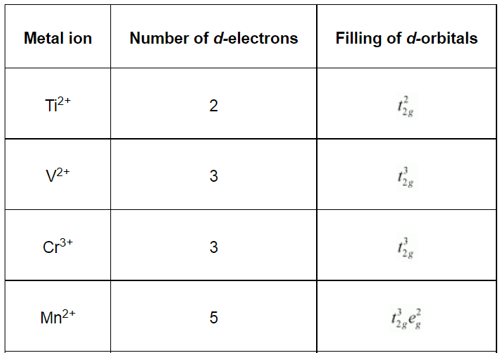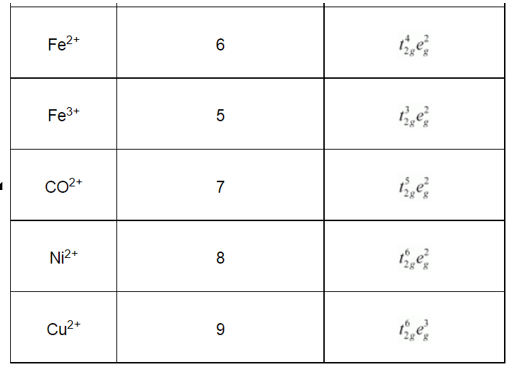Question 37: Comment on the statement that elements of the first transition series possess many properties different from those of heavier transition elements.

Solution. The properties of the elements of the first transition series differ from those of the heavier transition elements in many ways.

(i) The atomic sizes of the elements of the first transition series are smaller than those of the heavier elements (elements of $2^{\text {nd }}$ and $3^{\text {rd }}$ transition series).

However, the atomic sizes of the elements in the third transition series are virtually the same as those of the corresponding members in the second transition series. This is due to lanthanoid contraction.

(ii) $+2$ and $+3$ oxidation states are more common for elements in the first transition series, while higher oxidation states are more common for the heavier elements.

(iii) The enthalpies of atomisation of the elements in the first transition series are lower than those of the corresponding elements in the second and third transition series.

(iv) The melting and boiling points of the first transition series are lower than those of the heavier transition elements. This is because of the occurrence of stronger metallic bonding (M−M bonding).

(v) The elements of the first transition series form low-spin or high-spin complexes depending upon the strength of the ligand field. However, the heavier transition elements form only low-spin complexes, irrespective of the strength of the ligand field.

Question 38: What can be inferred from the magnetic moment values of the following complex species?

Example Magnetic Moment (BM)

$\mathrm{K}_{4}\left[\mathrm{Mn}(\mathrm{CN})_{6}\right] 2.2$

$\left[\mathrm{Fe}\left(\mathrm{H}_{2} \mathrm{O}\right)_{6}\right]^{2+} 5.3$

$\mathrm{K}_{2}\left[\mathrm{MnCl}_{4}\right] 5.9$

Solution. Magnetic moment $(\mu)$ is given as $\mu=\sqrt{n(n+2)}$.

For value $n=1, \mu=\sqrt{1(1+2)}=\sqrt{3}=1.732$.

For value $n=2, \mu=\sqrt{2(2+2)}=\sqrt{8}=2.83$.

For value $n=3, \mu=\sqrt{3(3+2)}=\sqrt{15}=3.87$.

For value $n=4, \mu=\sqrt{4(4+2)}=\sqrt{24}=4.899$.

For value $n=5, \mu=\sqrt{5(5+2)}=\sqrt{35}=5.92$.

(i) $\mathrm{K}_{4}\left[\mathrm{Mn}(\mathrm{CN})_{6}\right]$

For in transition metals, the magnetic moment is calculated from the spin-only formula. Therefore,

$\sqrt{n(n+2)}=2.2$

We can see from the above calculation that the given value is closest to $n=1$. Also, in this complex, Mn is in the $+2$ oxidation state. This means that Mn has 5 electrons in the $d$-orbital.

Hence, we can say that $\mathrm{CN}^{-}$is a strong field ligand that causes the pairing of electrons.

(ii) $\left[\mathrm{Fe}\left(\mathrm{H}_{2} \mathrm{O}\right)_{6}\right]^{2+}$

$\sqrt{n(n+2)}=5.3$

We can see from the above calculation that the given value is closest to $n=4$. Also, in this complex, Fe is in the $+2$ oxidation state. This means that Fe has 6 electrons in the $d$-orbital.

Hence, we can say that H2O is a weak field ligand and does not cause the pairing of electrons.

(iii) $\mathrm{K}_{2}\left[\mathrm{MnCl}_{4}\right]$

$\sqrt{n(n+2)}=5.9$

We can see from the above calculation that the given value is closest to $n=5$. Also, in this complex, Mn is in the $+2$ oxidation state. This means that Mn has 5 electrons in the $d$-orbital.

Hence, we can say that $\mathrm{Cl}^{-}$is a weak field ligand and does not cause the pairing of electrons.